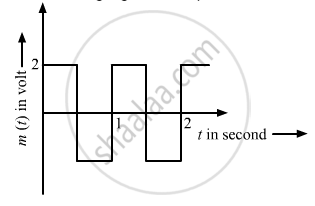# The Carrier Wave is Represented by C ( T ) = 5 Sin ( 10 π T ) a Modulating Signal is a Square Wave as Shown. Determine Modulation Index. - Physics

The carrier wave is represented by

$C(t) = 5 \sin(10\pi t)$
A modulating signal is a square wave as shown. Determine modulation index.#### Solution

Modulation index ($\mu$) is the ratio of the amplitude of the modulating signal to the amplitude of the carrier wave. The generalised equation of a carrier wave is given below: $c(t) = A_c \sin \omega_c t$

The generalised equation of a modulating wave is given below:

$c_m (t) = A_c \sin \omega_c t + \mu A_c \sin \omega_m t\sin \omega_c t$

Here,

$\mu$ is defined as $\frac{A_m}{A_c}$ .
On comparing this with the equations of carrier wave and modulating wave, we get:
Amplitude of modulating signal,
$A_m = 2 V$
Amplitude of carrier wave,
$A_c = 5 V$
∴ $\mu = \frac{A_m}{A_c} = \frac{2}{5} = 0 . 4$
Concept: Modulation and Its Necessity
Is there an error in this question or solution?